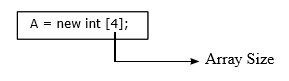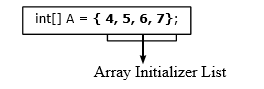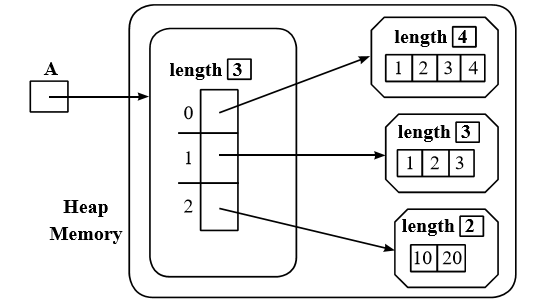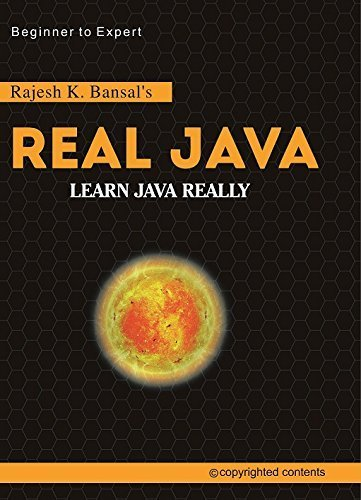### Array

Array is an indexed collection of finite number of homogenous data elements. Homogenous means that all the elements are of same type. Each array element is assigned an index value that starts from zero. The size of array is specified once and can not be changed later.

Actually, in Java, arrays are objects. An array in Java is created dynamically. An array variable does not store any value, basically it is a reference variable that points to array object. This array object can be of any type i.e. primitive or reference type.

An array object can contain a number of variables, it depends upon the size of array specified while constructing.The number of variables may be zero, in this case, the array is said to be empty.

The variables contained in array object are without names so they are referenced using index values. These variables are called components of the array. All components have same type, called component type of the array. Each array object consists of a final, int variable i.e. length, it consists of the size of an array.

### Creating Array

Array can be created using an array creation expression and array initializer list.
1. Array Creation Expression:
An array creation expression specifies the array variable, data type and array size with new operator, as shown in the following statement:The above statement creates an array object in heap memory. This array object will have four locations that are initialized to zero by default, as shown below:2. Array Initializers List:
By using array initializer list, an array can be declared, created and initialized by writing a single statement, shown as follows:### Passing Array as Argument

Arrays are passed by-reference. When an array is passed as an argument, the reference of array object is passed actually. The contents of an array can be changed inside the called method, however, we work on actual array directly not on a copy of array elements.

The following program consists of sorting() method , which receives reference of an array i.e. m from main() method, the sorting() method is called with m as parameter in line number:12.

```public class Sort
{
public static void main(String[] args)
{
int m[]={34,25,99,28,14};
int i;
System.out.println("Before Calling Method:");
for(i=0;i<m.length;i++)
{
System.out.print(m[i]+" ");
}
new Sort().sorting(m);//array passed by-reference
System.out.println(“\nAfter Calling Method:”);
for(i=0;i<m.length;i++)
{
System.out.print(m[i]+” “);
}
}
void sorting(int mr[])
{
int i,j,temp;
for(i=0;i<mr.length;i++)
{
for(j=0;j<mr.length-1-i;j++)
{
if(mr[j]>mr[j+1])
{
temp=mr[j];
mr[j]=mr[j+1];
mr[j+1]=temp;
}
}
}
}
}
```

Output: Before Calling Method: 34 25 99 28 14
After Calling Method: 14 25 28 34 99

### Anonymous Array

It is easy to create array using array creation expression and array initializer list, but array initialized once, can not be reinitialized later, unless you create the new array. This is where the anonymous array is used. Using anonymous array, existing array can be reinitialized to a new set of values, for example:

```1. int A[] = {4, 7, 8, 6};
2. A = new int[] {1, 2, 3, 9, 5};  // Anonymous Array. ```

In line number:2, array A is reinitialized with new values. But, keep in mind that while creating anonymous array, subscripts should be blank, means specifying length is invalid.

Anonymous array can also be used to pass unnamed arrays to methods, when you do not want to deﬁ ne a local variable for array to be passed as parameter, as shown in program below:

```// AnmsPass.java
class AnmsPass
{  public static void main(String[] args)
{	double avg=getAverage(new double[]{10.2,6.8,7,5,3});
System.out.println(“Average:”+avg);
}
static double getAverage(double[] data)
{	int i;
double sum=0;
for(i=0;i<data.length;i++)
sum=sum+data[i];
return sum/data.length;
}
}

```

### Multidimensional Array

In Java, a multidimensional array is an array of arrays. A multidimensional array can be declared as follows:
Syntax:
`<data type> [][].....[] <name of array> `
The above can also be written as follows:
` <data type>< name of array> [][].....[];`
Number of pairs of square brackets[], indicate, the number of dimensions, a 2-dimensional array variable can be declared using the following methods:The above statements just create a reference variable, which can refer to an array object consisting an array of object references.

A two-dimensional array can be constructed using the following code:
`int[][]A = new int;`
The above statement creates a reference variable A, which refers to an array of two elements, where each element further refers to an array of three elements, that are initialized to zero by default, as shown below:A two-dimensional array can also be created using initializer lists. As shown follows:
```int [][]B ={{1, 2, 3},
{4, 5, 6},
{7, 8, 9} };```
```int [][]D ={{5, 7, 8},
new int [] {8, 9, 10},
{1, 1, 1} };```
For example:
```class TwoD
{
public static void main(String[] args)
{	    int[][]D= {
{2,4,6},
new int[]{3,7,9},
{1,1,1}
};
System.out.println(“Output”);
for (int i = 0; i < D.length; i++)
{
for (int j = 0; j < 3; j++)
{
System.out.print(D[i][j]+”  “);
}
System.out.println();
}
}
} ```

### Jagged Array

In jagged array, each row of multidimensional array may contain different number of columns. It can be created using the following methods:
1. Initializer list
2. Array Creation Expression
1. Using Array Initializer List
```int A[] [] = {
{1, 2, 3, 4},
{1, 2, 3},
{10, 20}
}```
It is represented in memory as shown follows:2. Array Creation Expression To Construct 2-D Array:
```int [ ] [ ] A = new int  [ ];
A = new int[ ] {10, 20, 30};
A = new int;
A = new int[ ] {40, 50, 60};```
Implementation:
```class Jagged
{
public static void main(String[] args)
{	int[][] Z=new int[];
Z=new int[]{10,20,30};
Z=new int;
Z=new int[]{40,50,60};
System.out.println(“Output...”);
for(int i = 0; i < Z.length; i++)
{
for(int j = 0; j < Z[i].length; j++)
{
System.out.print(Z[i][j]+” “);
}
System.out.println();
}
}
}```

 About the Author Rajesh K. Bansal (SCJP-Sun Certified Java Programmer) 20 Years experience in Training & Development. Founder of realJavaOnline.com, loves coding in Java(J2SE, J2EE), C++,PHP, Python, AngularJS, Android,MERN Stack(MongoDB,Express,ReactJS,NodeJS). If you like tutorials and want to know more in depth about Java , buy his book "Real Java" available on amazon.in. #Email : bcebti@gmail.com #Contact : 98722-46056
##### Available on AmazonUnder the guidance of Founder & Author of "realJavaOnline.com". M:9872246056